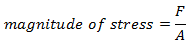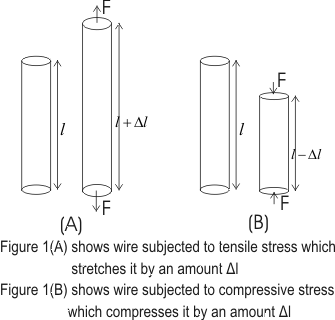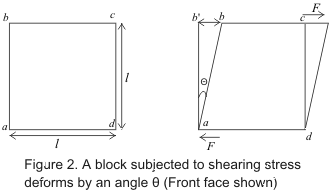# Elasticity

## 1. Introduction

• We know that any solid body has definite shape and size and more or less all solid bodies can be deformed by suitable application of forces.
• Forces producing deformation in any solid body can bring about changes in length , volume or shape of the body and the body is said to be strained or deformed.
• When deforming forces are removed the body tends to recover it's original condition.
• This property of material body to regain it's original condition, on removal of deforming forces is called ELASTICITY.
• When a solid body is deformed then it's constituents i.e., atoms or molecules gets displaced from their equilibrium position causing a change in inter-atomic or intermolecular distances.
• Again when the deforming forces are removed inter-atomic forces drives atoms or molecules back to their original equilibrium position. This way body regains it's original shape and size.

• DEFINITIONS
(a) Elastic forces:- The forces developed inside the body when deformed , tending to restore it's original shape are called elastic forces.
(b) Perfectly elastic bodies:- Bodies which which can recover their original condition completely on removal of deforming forces are called perfectly elastic bodies.
(c) Plastic bodies:- Bodies which does not show any tendency to recover their original condition on removal of deforming forces are called plastic bodies.

• There are no perfectly elastic or plastic bodies and actual bodies lie between two extremes.
• Nearest approach to perfectly elastic body is a quartz fiber and phosphor bronze (an alloy of copper containing 4% to 10% tin, 0.05% to 1% phosphorus)and an example of perfectly plastic body is putty.

Here is a good video explaining about Elastic and Plastic Deformation along with demonstration

## 2. Stress

• We know that when deforming forces acts on a body, forces of internal reaction develops inside the body which tends to restore the body to it's original position.
• These internal forces developed are equal in magnitude of deforming forces and acts in direction opposite to these externally applied deforming forces.
• Stress is this restoring force applied per unit area set up inside the body and is measured by the magnitude of deforming force acting on unit area with in the elastic limits of the body.
• Thus,where F is the force applied and A is the area of cross-section of the body.
• S.I. unit of stress is Nm-2 or pascal (Pa). In C.G.S. system it's unit is dynes/cm2.
• Dimensional formula for stress is [ML-1T-2].
• Let us now find out the difference between stress and pressure since they both have same formula and same dimensions
Difference between pressure and stress
Pressure Stress
Pressure is always normal to the area Stress can be normal or tangential
Always compressive in nature May be compressive or tensile in nature
• Stress are of two types
(a) Normal stress:-
• If elastic forces developed are perpendicular to the area of cross section of the body then the stress developed is known as normal stress.
• The stress is always normal in case of change in length of wire or in case of change in volume of body shown below in figure• The normal stress are of two types, tensile and compressive stress, accordingly as there is a increase or decrease in length or volume of body on application of force.
• Normal stress occurs only in solids and comes in picture when one of the three dimensions that is length, breadth or height is much greater than other two. In such a case when deforming force is applied parallel to the length it then causes increase in length.

• Bulk or volume stress
There is another type of stress called Bulk or Volume stress. It occurs in solids, liquids or gases. In case of fluids we can only found bulk stress. It produces change in volume and density while shape of the liquid remains the same. In this case deforming force is applied normal to surface at all points. It is equal to change in pressure because change in pressure is responsible for change in volume.
(b) Tangential or shearing stress:-
• Tangential or shearing developed in a body when elastic restoring forces are parallel to the cross-sectional area of the body as shown below in figure 2.• Thus when deforming force acts tangentially over an area the body gets sheared through a certain angle.
• Tangential stress comes into picture when there is a relative displacement between various layers of solid. Here deforming force is applied tangential to one of the faces as an be seen in the figure. This type of stress produces change in shape but volume remains the same.